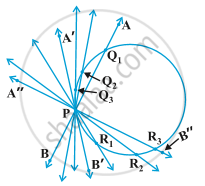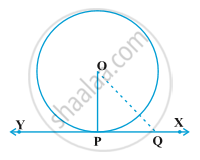Share

# Tangent to a Circle

#### description

Theorem - The tangent at any point of a circle is perpendicular to the radius through the point of contact.

#### notes

In the previous section, you have seen that a tangent to a circle is a line that intersects the circle at only one point.

1) A circle can only have one tangent at a point of the cirlce.But the circle is made of infinite numbers of points therefore a circle can have infinitely many tangents. But it can only have one tangent at one point.

#### theorem

Theorem1- The tangent at any point of a circle is perpendicular to the radius through the point of contact.Given: A circle with centre O, a tangent XY at the point of contanct P.

To prove: OP ⊥ XY.

Construction: Take a point Q, other than P or XY. Jion OQ.

Proof: Q lies on the tangent XY.

Q lies outside the circle.

Let OQ cuts the circle at R.

OR < OQ (a part is less than a whole)

But, OR=OP (radii of the same circle)

So, OP < OQ

Thus, OP is shorter than any line segment joining O to any point on XY other than point P.

Therefore, OP is the shortest distance between O and line segment XY.

But, the shortest distance between a point and a line segment is the perpendicular distance.

Therefore, OP is perpendicular to XY.

### Shaalaa.com

Line Tangent to a Circle is Perpendicular to the Radius [00:04:35]
S
2
0%

S